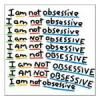# Search the Community

Showing results for tags 'drainable'.

• ### Search By Tags

Type tags separated by commas.

### Forums

• News & Announcements
• News
• Project Zomboid
• General Discussions
• Bug Reports
• PZ Support
• PZ Multiplayer
• PZ Community & Creativity
• PZ Suggestions
• PZ Modding
• Tutorials & Resources
• Mods
• Items
• Mapping
• Mod Ideas and Requests
• General Games Development
• Indie Scene
• Other Discussions
• General Discussion
• Forum Games & Activities

• 0 Replies

• 0 Views

### Interests

Found 5 results

1.## Problem with making a item trowable and drainable at the same time.

So i made a mod that adds a torch to the game. https://steamcommunity.com/sharedfiles/filedetails/?id=1542069453 Its is basically a torch that emits light around you in a circle and it depletes overtime, now I want to be able to trow it to start a fire. Problem is I can't because it is a "drainable" item, when I change it to a "weapon" it is trowable but it doesnt get depleted anymore? how can I make it trowable and still get drained when its being used. In the picture you can see how i tried to resolve it and how its currently set up. by giving it 2 item types it turns into blooo
2.## Showing remaining amount of drainable items in UI after expanding tree

It rustles my jimmies that while the nutrition (hunger) for food and remaining condition of weapons is neatly displayed when you expand the item in inventory, it doesn't show the remaining amount of drainable items and water containers, making you having to hover on each item to figure out which item is partialy depleted and which is not. It's less problem for water as you can pour water from one container to another, it's really problematic with other items, if you want to take a item with more uses on you or use up the ones that are partially empty to gain some extra weight in containers, since you can't combine them (another feature I'd love to see BTW). I know the UI update is coming down the road (I think!), but would be nice to see that before it anyway
3.## battery Remaining uses/drainable level in lua?

So I am looking for any information on retrieving and altering the remaining durability of a battery in code. I'm not finding much documentation on their use. Or any mods that make use of them in order to see how it is done. By the looks of things, in a multiplayer game I can use a torch, deplete the battery partially. Extract the battery and it is returned to max life. From the look of the recipes, taking the battery out just seems to craft a brand new one. Though I may be mistaken. Does this mean storing the depletion of a battery is not implemented yet? (Build 27) I would be really happy for any information regarding retrieving or altering the life of a battery in lua. Also greetings! ~
4.## Drainable Item UseDelta chart (make-shift chart)

The use delta is the amount of uses that Drainable items have. The formula is 1 divided by delta number = Number of uses. Thank you 7Roses . I will make a complete and better chart at some point. Unless someone else wants to use a calculator or their head to make it for us. 1=1 .1 = 9 .2 = 5 .3 = .4 = .5 = .6 = .7 = .8 = .9 =.01 = .02 = ALOT .03 = 33 .04 = 24 .05 = 19 .06 = 16 .07 = 14 .08 = 12 .09 = 11 .10 = .11 = .12 = .13 = .14 = .15 = 6 .16 = .17 = .18 = .19 = .20 = 5 .21 = .22 = .23 = .24 = .25 = 4 .26 = .27 = .28 = .29 = .30 = .31 = .32 = .33 = .34 = .35 = .36 = .37 = .38 = .39 = .40 = .41 = .42 = .43 = .44 = .45 = .46 = .47 = .48 = .49 = .50 = .51 = .52 = .53 = .54 = .55 = .56 = .57 = .58 = .59 = .60 = .001 = .002 = .003 = .004 = .005 = .006 = .007 = .008 = .009 = .010 = .011 = .012 = .013 = .014 = .015 = .016 = .017 = .018 = .019 = .020 = .021 = .022 = .023 = .024 = .025 = .026 = .027 = .028 = .029 = .030 = .031 = .032 = .033 = .034 = .035 = .036 = .037 = .038 = .039 = .040 = .041 = .042 = .043 = .044 = .045 = .046 = .047 = .048 = .049 = .050 = .051 = .052 = .053 = .054 = .055 = .056 = .057 = .058 = .059 = .060 =
5. I would like to see the pens and pencils have a UseDelta and be Drainable, with the pencil running out quick and the pen lasting longer. The only way to do this is to make the base vanilla pen and pencil into Drainable items instead of weapons. You could then add a weaponization crafting recipe using a ripped sheet or something and the pen or pencil to make it a weapon, once you make it a weapon you cannot change it back nor can you write with it.
×
×
• Create New...